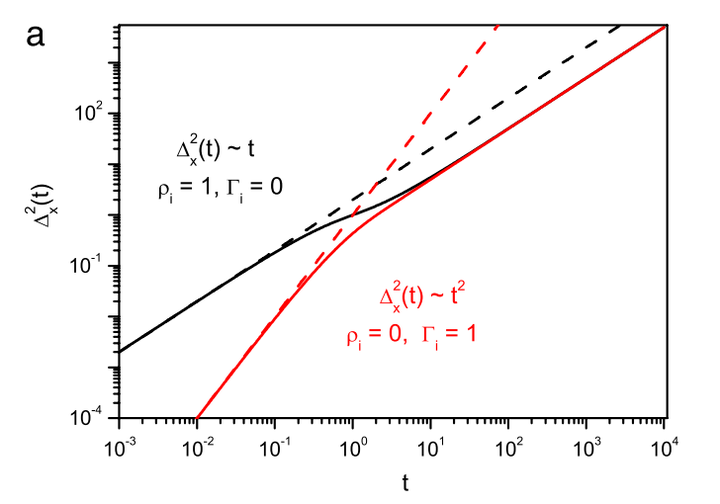# Solutions for a sorption process governed by a fractional diffusion equation### Abstract

We investigate a sorption process where one substance spreads out through another having possibility of chemical reaction between them. So as to describe this process, we have considered the bulk dynamics governed by a fractional diffusion equation, where the reaction term may describe an irreversible or a reversible process. This reaction term represents a generalization of the first order kinetic equation taking memory effects into account. The analytical solutions for the mean square displacement, survival probability and probability density of the particles we have obtained show a rich class of behaviors connected to anomalous diffusion.

Type